## Divergence Theorem

A.k.a. Gauss's Theorem. Letbe a region in space with boundary. Then(1)

Letbe a region in the plane with boundary.(2)

If the Vector Fieldsatisfies certain constraints, simplified forms can be used. Ifwhere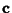is a constant vector, then(3)

But(4)

so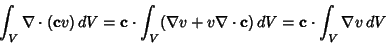(5)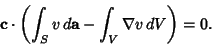(6)

But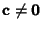, and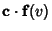must vary with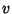so thatcannot always equal zero. Therefore,(7)

If, whereis a constant vector, then(8)

Arfken, G. Gauss's Theorem.'' §1.11 in Mathematical Methods for Physicists, 3rd ed. Orlando, FL: Academic Press, pp. 57-61, 1985.